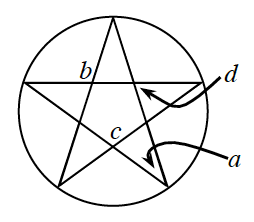### Home > INT2 > Chapter 12 > Lesson 12.2.2 > Problem12-92

12-92.The figure at right is a pentagram inscribed in a circle.  A pentagram is a $5$-pointed star that has congruent angles at each of its outer vertices.

1. Use the fact that all pentagrams can be inscribed in a circle to calculate the measure of angle $a$ at right.

$36°$

2. What are the measures of angles $b, c,$ and $d$?  Show your work.

$∠b$ is equivalent to $∠c$